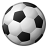Announcements
MAINTENANCE ALERT: Dec. 12th starting 9 AM CET. Community will be read-only. GET DETAILS
cancel
Showing results for
Did you mean:Creator

## Show 12 months of value, when selecting a month

Afternoon team, hope you're all well and happy friday.

Quick query I hopeI've attached an example qvw, where I'm trying to achieve a rolling 12 month total when you select a month.

As per my attached, currently if you select say 2018 Nov, this will then change the bar chat to show 2018 Nov and the previous 11 individual month totals. So as a month calculation, this works perfect to show each individual month across the last 12 months.

However, i'm a little stuck as to how I can have each one of those 12 categories, showing a 12 month total.

For example select 2018 Nov, this will show data between 2018 Nov - 2017 Dec (12 month total)

Then the category next to it being 2018 Oct, will show data between 2018 Oct - 2017 Nov and so on.

Hope this makes sense and can help me piece this together

Many thanks

1 Solution

Accepted SolutionsMVP

May be try this

=RangeSum(Above(Sum({<[Period_Sort]=,Month= ,Year_YYYY= ,Quarter_QQ= , Period_YYYY_Month=,

Customer={"=round(Sum({<[Period_Sort]={"">=\$(=max([Period_Sort])-11)<=\$(=max([Period_Sort]))""},Month= ,Year_YYYY= ,Quarter_QQ= ,Period_YYYY_Month =>}Revenue))<=\$(vMinLimit)

or

round(Sum({<[Period_Sort]={"">=\$(=max([Period_Sort])-11)<=\$(=max([Period_Sort]))""},Month= ,Year_YYYY= ,Quarter_QQ= ,Period_YYYY_Month= >}Revenue))>=\$(vMaxLimit)"}>} Revenue), 0, 12))

* Avg({<[Period_Sort]={">=\$(=max([Period_Sort])-11)<=\$(=max([Period_Sort]))"},Month= ,Year_YYYY= ,Quarter_QQ= , Period_YYYY_Month=>} 1)

2 RepliesMVP

May be try this

=RangeSum(Above(Sum({<[Period_Sort]=,Month= ,Year_YYYY= ,Quarter_QQ= , Period_YYYY_Month=,

Customer={"=round(Sum({<[Period_Sort]={"">=\$(=max([Period_Sort])-11)<=\$(=max([Period_Sort]))""},Month= ,Year_YYYY= ,Quarter_QQ= ,Period_YYYY_Month =>}Revenue))<=\$(vMinLimit)

or

round(Sum({<[Period_Sort]={"">=\$(=max([Period_Sort])-11)<=\$(=max([Period_Sort]))""},Month= ,Year_YYYY= ,Quarter_QQ= ,Period_YYYY_Month= >}Revenue))>=\$(vMaxLimit)"}>} Revenue), 0, 12))

* Avg({<[Period_Sort]={">=\$(=max([Period_Sort])-11)<=\$(=max([Period_Sort]))"},Month= ,Year_YYYY= ,Quarter_QQ= , Period_YYYY_Month=>} 1)Creator
Author

Hi Sunny, very helpful as always and this seems to work wondersmay i ask, what does that last paragraph of code below actually do?

So by adding in an average and leaving a 1 at the end?

Cheers

* Avg({<[Period_Sort]={">=\$(=max([Period_Sort])-11)<=\$(=max([Period_Sort]))"},Month= ,Year_YYYY= ,Quarter_QQ= , Period_YYYY_Month=>} 1)Community Browser